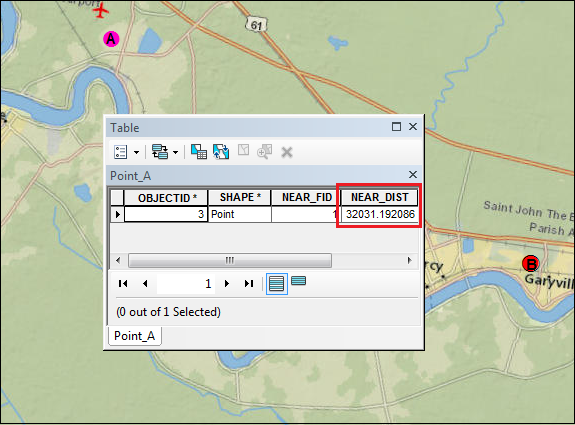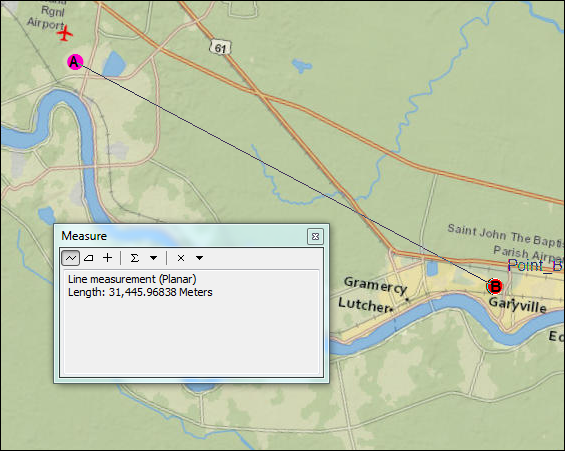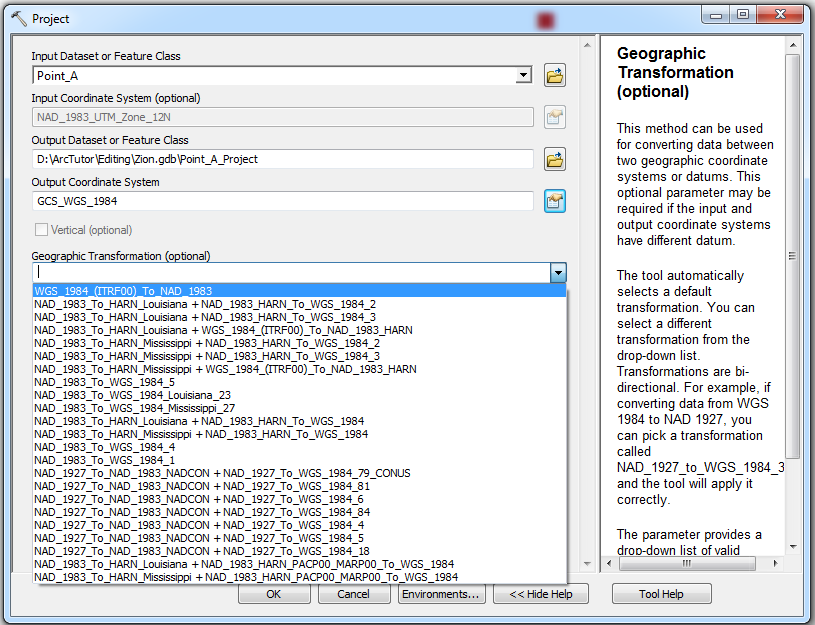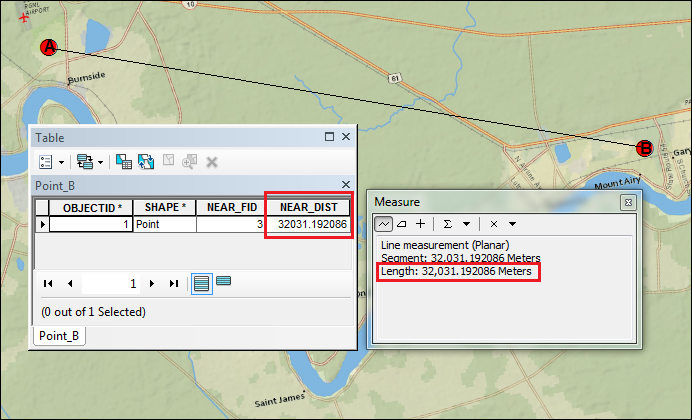English

# Problem: The Near tool returns different results than the Measure tool

## Description

When calculating the distance between a given feature and the closest feature in another layer or feature class using the Near tool, the results returned are different when compared to the distance measured between the same features using the Measure tool.

The following are examples of the results returned when calculating the distance between feature A and feature B using the Near tool and the Measure tool. In the following images, the measurement taken between points A and B using the Near tool shows a distance of 32,031.192086 meters, while the same distance, when measured using the Measure tool, shows a distance of 31,445.96838 meters.## Cause

The discrepancy occurs due to the difference in the coordinate system used between the dataset and the data frame. The Near tool relies on the input dataset coordinate system, whereas the Measure tool relies on the data frame coordinate system.

## Solution or Workaround

To ensure the Near tool is returning the same distance as the Measure tool, the coordinate system used by the dataset and the data frame must be the same. Depending on whether the data frame or the dataset has the correct coordinate system, use one of the following options to solve this issue:

Match the dataset coordinate system to the data frame coordinate system

For cases in which the data frame has the correct coordinate system, match the coordinate system of the dataset to the data frame coordinate system using the Project tool:

1. In the Catalog window, navigate to Data Management Tools > Projections and Transformations > Project.
2. Choose the dataset as the Input Dataset or Feature Class. The Input Coordinate System parameter is automatically set with the original coordinate system of the dataset.
3. Set a name and location for the Output Dataset or Feature Class.
4. In Output Coordinate System, browse for the data frame coordinate system to assign to the output dataset.
5. In the Geographic Transformation parameter, a transformation is automatically selected by default. To select a different transformation, click the drop-down arrow and select the suitable geographic transformation of the coordinate systems. The drop-down list only appears when a geographic or datum transformation is required.6. Check the Preserve Shape check box to ensure the output features are accurately located in the new projection. This option is only available when a geographic or datum transformation is involved.
7. Set a value for the Maximum Offset Deviation parameter to control the maximum distance the projected feature can be offset from its projected location.
8. Click OK.

Match the data frame coordinate system to the dataset coordinate system

For cases in which the dataset has the correct coordinate system, change the coordinate system of the data frame: Changing the data frame's coordinate system.

The figure below is an example of the results of the two tools when measuring the distance between two points when the dataset and data frame use the same coordinate system. Both tools display the same distance.```Note:
In cases where both the dataset and data frame have the wrong coordinate system, use the same steps to change the coordinate system of the dataset and data frame to the most accurate coordinate system. Refer to the following document to determine which coordinate system is the most suitable for a dataset: How To: Select a suitable map projection or coordinate system.```